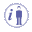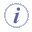# List

List methods are used to manage lists of parameters, pads ... They enable you to create lists, to add items to the list, to remove items from the list, to retrieve values from the list, to move elements of the list to another position, to filter, and to copy the content of a list into another one.The functions described below are available in the Formula, the Rule and in the Action editors.
• List->Size () : Integer
Method used to return the number of items contained in the list.
• List->GetItem (Index: Integer) :ObjectType
Method used to retrieve a value/item from the list. (Index from 1).
• Copy (List: List) : List
Method used to copy the content of a list and paste it in another list.
• List (Next: ObjectType, ...): List
Method used to create a list.
 `List(Item1, Item2, Item3)`
• List->Sum (): Real
Computes the sum of the items contained in the list. Available for integers and real numbers only.
• List->IndexOf (Element: ObjectType, StartIndex:Integer):Integer
Returns the first index of a list item. The item is searched for from the start index.The functions described below are available in the Action editor only.
• List->AddItem (Object: Objecttype, Index: Integer):VoidType
Method used to add an item to the list. If the index is equal to 0, the new item is added at the end of the list. If the index is equal to 1, the new item is inserted into the list at the location indicated by the index, meaning that the existing item is replaced with the new one and is therefore removed from the list. It is recommended to use the InsertItem method.
 ``` let list (List) list->AddItem(PartBody\Hole.2 ,1) list->AddItem(PartBody\Hole.3 ,2) Message("#",list.Size())```
• List->InsertItem (Object:ObjectType, Index: Integer): VoidType
Method used to insert an item into the list. Where:
 Object is the object to insert into the list. Index is the location of the object to be inserted into the list.
• List->Append (Object:ObjectType)
Method used to add an item at the end of the list. Where:
 Object is the object to insert into the list.
• List->SetItem (Object:ObjectType, Index: Integer): VoidType
Method used to replace an item in the list. Where:
 Object is the object to insert into the list. Index is the location of the object to be replaced in the list. In this case, the object to be replaced is deleted.
• List->RemoveItem (Index: Integer) :VoidType
Method used to remove an item from the list.
• List->RemoveAll(): VoidType
Empties the list.
• List->ReorderItem (Current: Integer, Target: Integer ) :ObjectType
Method used to move an element of the list referenced by its position to a new position.
• List->Compute(Operation: String, Type: String, Expression: String, Result: out UndefinedType): VoidType
Function used to compute the result of an operation performed on the attributes supported by the features contained in the list.
Syntax: List->Compute ("+","","",result)
Example: List.1 .Compute("+","","",Length.1)
Where:
 List.1 is the name of the list on which the calculation will be performed + is the operator used. (Supported operators are: +, min, and max.) The first "" is the type of the list items used for the calculation (to calculate the diameter, the type to be indicated is Hole, to calculate the volume, the type to be indicated is Solid) The second"" stands for the list items. Note that the type of the items contained in the list must be identical. Length.1 is the output parameter.Note that this method is available for Actions, Reactions and in Knowledge Expert.
• List->Apply (Type:String, Expression: String): VoidType
Applies a given expression to the objects of a list that are of a given type.

Example:

 //Finds all rule bases and executes them ```Expression1 (P: #In PartFeature) {```  /* Finding a value */ ```let L (List) L = P->Query("Rulebase","") L->Apply ("RuleBase","x->Update()") }```
• List->Filter(Type:String, Condition: String): List
Method used to filter a list of objects by extracting the objects that fulfill a Boolean expression.
This method has the following signature:
`List.Filter(String TypeName,String Expression) : List`
 `TypeName` is the type of the objects that the user wants to extract (in can be "". In this case, no filtering is done on types) The second string Expression corresponds to the Boolean expression that must fulfill the objects of this given type. In this expression "x" is used as the variable name of type TypeName. This string can be equal to "". In this case, no expression is checked.

Example:
```I = (List->Filter("Hole","x.Diameter > 3mm")).Size() I = (List->Filter("Hole","")).Size()```